## 例子### 答案：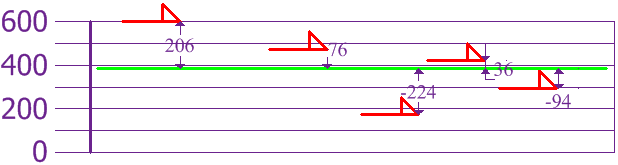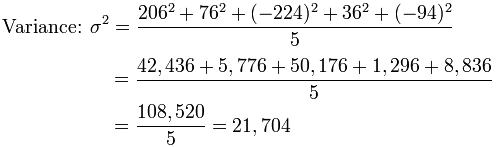标准差 σ = √21,704 = 147.32…… = 147 （到最近的毫米）## 可是……如果数据是样本数据

• 对象总体：在求方差时除以 N（如上）
• 样本：在求方差时除以 N-1

## 公式

 “对象总体标准差”：“样本标准差“：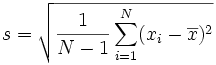### *脚注：为什么要求差的平方？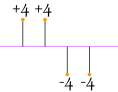4 + 4 − 4 − 44 = 0|4| + |4| + |−4| + |−4|4 = 4 + 4 + 4 + 44 = 4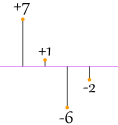|7| + |1| + |−6| + |−2|4 = 7 + 1 + 6 + 24 = 4√(42 + 42 + 42 + 424) = √(644) = 4√(72 + 12 + 62 + 224) = √(904) = 4.74…

From:

https://www.shuxuele.com/data/standard-deviation.html#Top